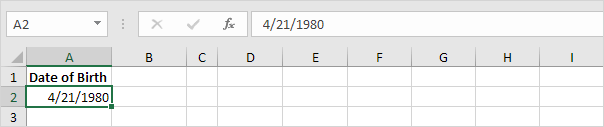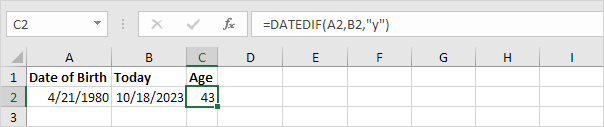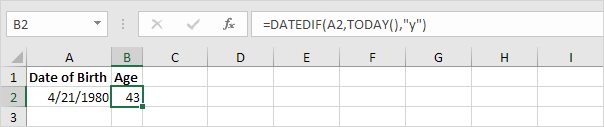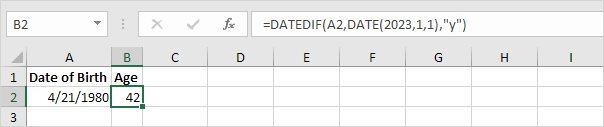# Calculate Age

To calculate the age of a person in Excel, use DATEDIF and TODAY. The DATEDIF function has three arguments.

1. Enter the date of birth into cell A2.2. Enter the TODAY function into cell B2 to return today's date.3. The DATEDIF function below calculates the age of a person.Note: fill in "y" for the third argument to get the number of complete years between the date of birth and today's date.

4. Calculate the age of a person without displaying today's date.5. Calculate the age of a person on a specific date.Note: the DATE function accepts three arguments: year, month and day.

6. The age formula below calculates the age of a person in years, months and days.Note: fill in "ym" for the third argument to ignore years and get the number of months between two dates. Fill in "md" for the third argument to ignore months and get the number of days between two dates. Use the & operator to join strings.

Go to Next Chapter: Text Functions